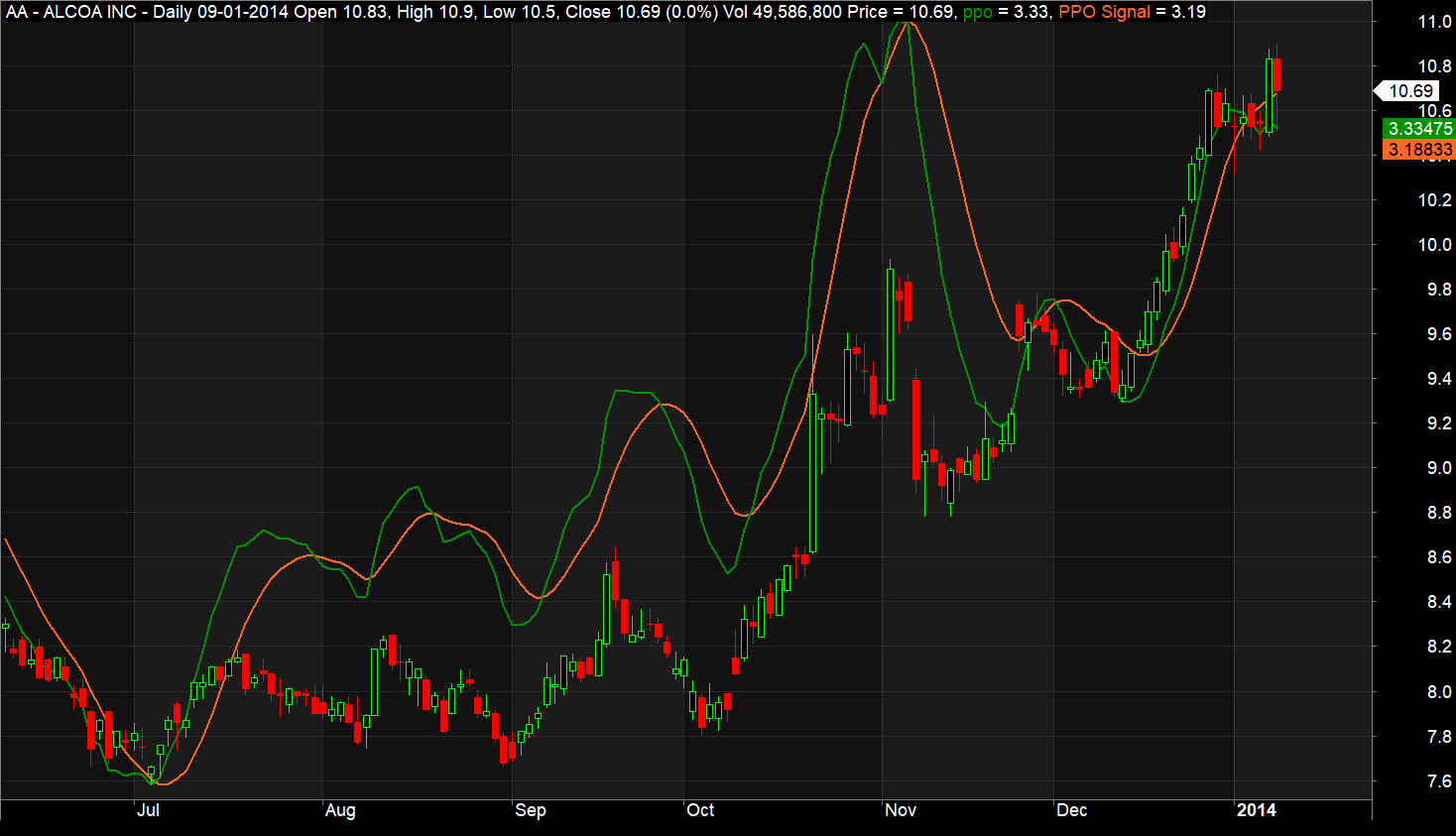# Percentage Price Oscillator AFL Code

Percentage Price Oscillator, also known as PPO is another momentum indicator that indicates the percentage price difference between two moving averages. Mathematically it is the difference of shorter moving average and longer moving average divided by longer moving average.

PPO is very similar to MACD (Moving average convergence divergence) with the only variance that it measures the difference between shorter and longer moving averages in percentage (%) terms, in MACD this difference is measured in absolute dollar value. This makes it easier to compare the PPO values of different assets.

By default, the moving averages used for PPO calculation are 12-period and 26-period exponential moving averages, but traders generally adjust the period according to their convenience.

Similar to MACD, the PPO indicator also has an associated ‘signal’ line, which is the EMA value of PPO itself. The signal line moves slower than PPO. Also, the difference between PPO and signal line is termed as ‘PPO histogram’.

PPO is positive when a shorter period moving average is higher than a longer period moving average. A higher positive value of PPO indicates strong upside momentum. Similarly, PPO is negative when a longer period moving average is higher than a shorter period moving average. A lower negative value of PPO indicates strong downside momentum.

## Preferred Timeframe

The indicator works well in all the timeframes. But you should look at multiple timeframes for extra confirmation of the signal

Also Read: Acceleration Bands AFL Code

## Screenshot

Below is the screenshot of the percentage price oscillator plotted in an Amibroker chart. The green line represents PPO, and the red line represents a signal. These lines are plotted on their own scale.Percentage price oscillator AFL Code

### AFL Explanation

After plotting the chart title and candlestick chart, the PPO and signal line is calculated using the below formulas:

PPO = (26-period EMA / (26-period EMA​) × 100

Signal Line = 9-period EMA of PPO

Where

EMA = exponential moving average

Range = (High – Low) / (High + Low)/2

And then, these lines are plotted on a candlestick chart by using the standard Amibroker “plot” function.

Do you want to learn AFL coding from scratch and build your own profitable trading systems? Check out our best-selling Algorithmic trading Bootcamp course bundle.

### Long and Short Rules

The long and short rules are similar to MACD.

1. If the signal line crosses over PPO line, then go long
2. If the signal line crosses under PPO line, then go short
3. If PPO is above zero, it indicates uptrend otherwise downtrend
4. PPO can also be used to assess the technical divergence between price and indicator. For example- if price makes a higher high and PPO makes a lower high, then the bullish phase of the asset may be subsiding. And if price makes a lower low and PPO makes a higher low, then the bearish phase of the asset may come to an end soon

#### Disclaimer

All the AFL’s posted in this section are for learning purposes. Trading Tuitions does not necessarily own these AFL’s and we don’t have any intellectual property rights on them. We might copy useful AFL’s from public forums and post it in this section in a presentable format. The intent is not to copy anybody’s work but to share knowledge. If you find any misleading or non-reproducible content then please inform us at support@tradingtuitions.com